# NCERT Solutions For Class 10 Math Chapter – 6 Exercise – 6.6

NCERT Solutions For Class 10 Math Chapter – 6 Exercise – 6.6

Q1.  In figure 6.56 PS is the bisector of QPR of ∆PQR Prove that QS/SR = PQ/PR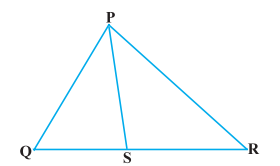Solution:-

∟1 = ∟2 (Alternate interior angle)   (I)

RT // SP and transversal QT intersects them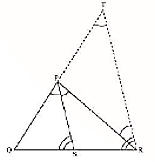∟3 = ∟4  (Corresponding angle)  (ii)

But ∟1 = ∟3 (Given)

∟2 = ∟4 ( From equation (I) and (ii)]

PT = PR ( Sides opposite to equal angles of a triangle are equal )

Now in ∆QRT ,

PS // RT ( By constructions)

QS / SR = PQ/PT ( By basic proportionally theorem)

QS/SR

= PQ/PR ( From equation (iii) ]

Q2. In fig 6.57 D  is a point on hypotenuse AC of ∆ABC , Such that BD altitude AC , DM altitude BC and

DN altitude AB. Prove that:

• DM2= DN.MC
• DN2= DM.AM

Solution:-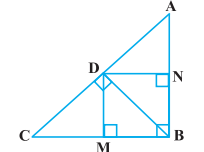Let us join Point D and B.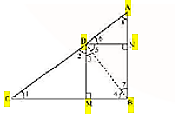Given,

BD ⊥AC, DM ⊥ BC and DN ⊥ AB

Now from the figure we have,

DN || CB, DM || AB and ∠B = 90 °

Therefore, DMBN is a rectangle.

So, DN = MB and DM = NB

The given condition which we have to prove, is when D is the foot of the perpendicular drawn from B to AC.

∴ ∠CDB = 90° ⇒ ∠2 + ∠3 = 90° ……………………. (i)

In ∆CDM, ∠1 + ∠2 + ∠DMC = 180°

⇒ ∠1 + ∠2 = 90° …………………………………….. (ii)

In ∆DMB, ∠3 + ∠DMB + ∠4 = 180°

⇒ ∠3 + ∠4 = 90° …………………………………….. (iii)

From equation (i) and (ii), we get

∠1 = ∠3

From equation (i) and (iii), we get

∠2 = ∠4

In ∆DCM and ∆BDM,

∴ ∆DCM ∼ ∆BDM (AA similarity criterion)

BM/DM = DM/MC

DN/DM = DM/MC (BM = DN)

⇒ DM2 = DN × MC

Hence, proved.

(ii) In right triangle DBN,

∠5 + ∠7 = 90° ……………….. (iv)

In right triangle DAN,

∠6 + ∠8 = 90° ………………… (v)

D is the point in triangle, which is foot of the perpendicular drawn from B to AC.

∴ ∠ADB = 90° ⇒ ∠5 + ∠6 = 90° ………….. (vi)

From equation (iv) and (vi), we get,

∠6 = ∠7

From equation (v) and (vi), we get,

∠8 = ∠5

In ∆DNA and ∆BND,

∴ ∆DNA ∼ ∆BND (AA similarity criterion)

AN/DN = DN/NB

⇒ DN2 = AN × NB

⇒ DN2 = AN × DM (Since, NB = DM)

Hence, proved.

1. In Figure, ABC is a triangle in which ∠ABC > 90° and AD ⊥ CB produced. Prove that

AC2= AB2+ BC2+ 2 BC.BD.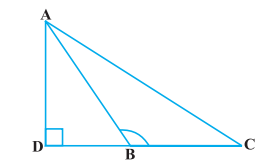Solution:

By applying Pythagoras Theorem in ∆ADB, we get,

AB2 = AD2 + DB2 ……………………… (i)

Again, by applying Pythagoras Theorem in ∆ACD, we get,

AC2 = AD2 + (DB + BC) 2

AC2 = AD2 + DB2 + BC2 + 2DB × BC

From equation (i), we can write,

AC2 = AB2 + BC2 + 2DB × BC

Hence, proved.

1. In Figure, ABC is a triangle in which ∠ ABC < 90° and AD ⊥ BC. Prove that

AC2= AB2+ BC2 – 2 BC.BD.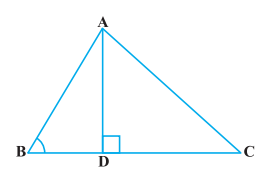Solution:

By applying Pythagoras Theorem in ∆ADB, we get,

We can write it as;

⇒ AD2 = AB2 − DB2 ……………….. (i)

By applying Pythagoras Theorem in ∆ADC, we get,

From equation (i),

AB2 − BD2 + DC2 = AC2

AB2 − BD2 + (BC − BD) 2 = AC2

AC2 = AB2 − BD2 + BC2 + BD2 −2BC × BD

AC2 = AB2 + BC2 − 2BC × BD

Hence, proved.

1. In Figure, AD is a median of a triangle ABC and AM ⊥ BC. Prove that :

(i) AC2 = AD2 + BC.DM + 2 (BC/2) 2

(ii) AB2 = AD2 – BC.DM + 2 (BC/2) 2

(iii) AC2 + AB2 = 2 AD2 + ½ BC2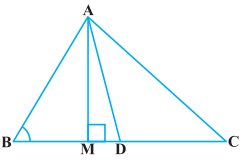Solution:

(i) By applying Pythagoras Theorem in ∆AMD, we get,

AM2 + MD2 = AD2 ………………. (i)

Again, by applying Pythagoras Theorem in ∆AMC, we get,

AM2 + MC2 = AC2

AM2 + (MD + DC) 2 = AC2

(AM2 + MD2 ) + DC2 + 2MD.DC = AC2

From equation(i), we get,

AD2 + DC2 + 2MD.DC = AC2

Since, DC=BC/2, thus, we get,

AD2 + (BC/2) 2 + 2MD.(BC/2) 2 = AC2

AD2 + (BC/2) 2 + 2MD × BC = AC2

Hence, proved.

(ii) By applying Pythagoras Theorem in ∆ABM, we get;

AB2 = AM2 + MB2

= (AD2 − DM2) + MB2

= (AD2 − DM2) + (BD − MD) 2

= AD2 − DM2 + BD2 + MD2 − 2BD × MD

= AD2 + BD2 − 2BD × MD

= AD2 + (BC/2)2 – 2(BC/2) MD

= AD2 + (BC/2)2 – BC MD

Hence, proved.

(iii) By applying Pythagoras Theorem in ∆ABM, we get,

AM2 + MB2 = AB2 ………………….… (i)

By applying Pythagoras Theorem in ∆AMC, we get,

AM2 + MC2 = AC2 …………………..… (ii)

Adding both the equations (i) and (ii), we get,

2AM2 + MB2 + MC2 = AB2 + AC2

2AM2 + (BD − DM) 2 + (MD + DC) 2 = AB2 + AC2

2AM2+BD2 + DM2 − 2BD.DM + MD2 + DC2 + 2MD.DC = AB2 + AC2

2AM2 + 2MD2 + BD2 + DC2 + 2MD (− BD + DC) = AB2 + AC2

2(AM2+ MD2) + (BC/2) 2 + (BC/2) 2 + 2MD (-BC/2 + BC/2) 2 = AB2 + AC2

2AD2 + BC2/2 = AB2 + AC2

1. Prove that the sum of the squares of the diagonals of parallelogram is equal to the sum of the squares of its sides.

Solution:

Let us consider, ABCD be a parallelogram. Now, draw perpendicular DE on extended side of AB, and draw a perpendicular AF meeting DC at point F.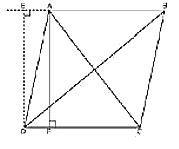By applying Pythagoras Theorem in ∆DEA, we get,

DE2 + EA2 = DA2 ……………….… (i)

By applying Pythagoras Theorem in ∆DEB, we get,

DE2 + EB2 = DB2

DE2 + (EA + AB) 2 = DB2

(DE2 + EA2) + AB2 + 2EA × AB = DB2

DA2 + AB2 + 2EA × AB = DB2 ……………. (ii)

By applying Pythagoras Theorem in ∆ADF, we get,

Again, applying Pythagoras theorem in ∆AFC, we get,

AC2 = AF2 + FC2 = AF2 + (DC − FD) 2

= AF2 + DC2 + FD2 − 2DC × FD

= (AF2 + FD2) + DC2 − 2DC × FD AC2

AC2= AD2 + DC2 − 2DC × FD ………………… (iii)

Since ABCD is a parallelogram,

AB = CD ………………….…(iv)

And BC = AD ………………. (v)

∠DEA = ∠AFD (Each 90°)

∴ ∆EAD ≅ ∆FDA (AAS congruence criterion)

⇒ EA = DF ……………… (vi)

Adding equations (i) and (iii), we get,

DA2 + AB2 + 2EA × AB + AD2 + DC2 − 2DC × FD = DB2 + AC2

DA2 + AB2 + AD2 + DC2 + 2EA × AB − 2DC × FD = DB2 + AC2

From equation (iv) and (vi),

BC2 + AB2 + AD2 + DC2 + 2EA × AB − 2AB × EA = DB2 + AC2

AB2 + BC2 + CD2 + DA2 = AC2 + BD2

1. In Figure, two chords AB and CD intersect each other at the point P. Prove that :

(i) ∆APC ~ ∆ DPB

(ii) AP . PB = CP . DP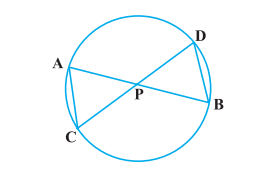Solution:

Firstly, let us join CB, in the given figure.

(i) In ∆APC and ∆DPB,

∠APC = ∠DPB (Vertically opposite angles)

∠CAP = ∠BDP (Angles in the same segment for chord CB)

Therefore,

∆APC ∼ ∆DPB (AA similarity criterion)

(ii) In the above, we have proved that ∆APC ∼ ∆DPB

We know that the corresponding sides of similar triangles are proportional.

∴ AP/DP = PC/PB = CA/BD

⇒AP/DP = PC/PB

∴AP. PB = PC. DP

Hence, proved.

1. In Fig. 6.62, two chords AB and CD of a circle intersect each other at the point P (when produced) outside the circle. Prove that:

(i) ∆ PAC ~ ∆ PDB

(ii) PA . PB = PC . PD.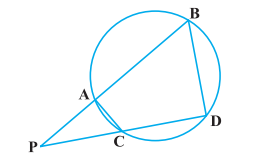Solution:

(i) In ∆PAC and ∆PDB,

∠P = ∠P (Common Angles)

As we know, exterior angle of a cyclic quadrilateral is ∠PCA and ∠PBD is opposite interior angle, which are both equal.

∠PAC = ∠PDB

Thus, ∆PAC ∼ ∆PDB(AA similarity criterion)

(ii) We have already proved above,

∆APC ∼ ∆DPB

We know that the corresponding sides of similar triangles are proportional.

Therefore,

AP/DP = PC/PB = CA/BD

AP/DP = PC/PB

∴ AP. PB = PC. DP

1. In Figure, D is a point on side BC of ∆ ABC such that BD/CD = AB/AC. Prove that AD is the bisector of ∠ BAC.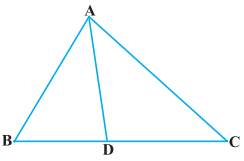Solution:

In the given figure, let us extend BA to P such that;

AP = AC.

Now join PC.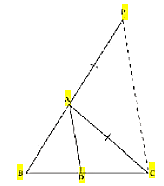Given, BD/CD = AB/AC

⇒ BD/CD = AP/AC

By using the converse of basic proportionality theorem, we get,

∠BAD = ∠APC (Corresponding angles) ……………….. (i)

And, ∠DAC = ∠ACP (Alternate interior angles) …….… (ii)

By the new figure, we have;

AP = AC

⇒ ∠APC = ∠ACP ……………………. (iii)

On comparing equations (i), (ii), and (iii), we get,

Therefore, AD is the bisector of the angle BAC.

Hence, proved.

1. Nazima is fly fishing in a stream. The tip of her fishing rod is 1.8 m above the surface of the water and the fly at the end of the string rests on the water 3.6 m away and 2.4 m from a point directly under the tip of the rod. Assuming that her string (from the tip of her rod to the fly) is taut, how much string does she have out (see Figure)? If she pulls in the string at the rate of 5 cm per second, what will be the horizontal distance of the fly from her after 12 seconds?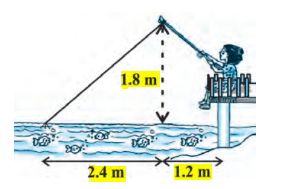Solution:

Let us consider, AB is the height of the tip of the fishing rod from the water surface and BC is the

horizontal distance of the fly from the tip of the fishing rod. Therefore, AC is now the length of the string.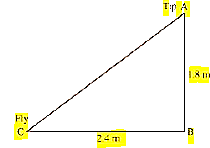To find AC, we have to use Pythagoras theorem in ∆ABC, is such way;

AC2 = AB2+ BC2

AB2 = (1.8 m) 2 + (2.4 m) 2

AB2 = (3.24 + 5.76) m2

AB2 = 9.00 m2

⟹ AB = √9 m = 3m

Thus, the length of the string out is 3 m.

As its given, she pulls the string at the rate of 5 cm per second.

Therefore, string pulled in 12 seconds = 12 × 5 = 60 cm = 0.6 m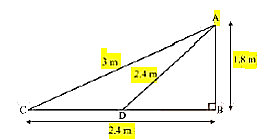Let us say now, the fly is at point D after 12 seconds.

Length of string out after 12 seconds is AD.

AD = AC − String pulled by Nazima in 12 seconds

= (32. In Fig. 6.57, D is a point on hypotenuse AC of ∆ABC, such that BD ⊥AC, DM ⊥ BC and DN ⊥ AB. Prove that: (i) DM2 = DN . MC (ii) DN2 = DM . AN.

Solution:

1. Let us join Point D and B.

Given,

BD ⊥AC, DM ⊥ BC and DN ⊥ AB

Now from the figure we have,

DN || CB, DM || AB and ∠B = 90 °

Therefore, DMBN is a rectangle.

So, DN = MB and DM = NB

The given condition which we have to prove, is when D is the foot of the perpendicular drawn from B to AC.

∴ ∠CDB = 90° ⇒ ∠2 + ∠3 = 90° ……………………. (i)

In ∆CDM, ∠1 + ∠2 + ∠DMC = 180°

⇒ ∠1 + ∠2 = 90° …………………………………….. (ii)

In ∆DMB, ∠3 + ∠DMB + ∠4 = 180°

⇒ ∠3 + ∠4 = 90° …………………………………….. (iii)

From equation (i) and (ii), we get

∠1 = ∠3

From equation (i) and (iii), we get

∠2 = ∠4

In ∆DCM and ∆BDM,

∴ ∆DCM ∼ ∆BDM (AA similarity criterion)

BM/DM = DM/MC

DN/DM = DM/MC (BM = DN)

⇒ DM2 = DN × MC

Hence, proved.

(ii) In right triangle DBN,

∠5 + ∠7 = 90° ……………….. (iv)

In right triangle DAN,

∠6 + ∠8 = 90° ………………… (v)

D is the point in triangle, which is foot of the perpendicular drawn from B to AC.

∴ ∠ADB = 90° ⇒ ∠5 + ∠6 = 90° ………….. (vi)

From equation (iv) and (vi), we get,

∠6 = ∠7

From equation (v) and (vi), we get,

∠8 = ∠5

In ∆DNA and ∆BND,

∴ ∆DNA ∼ ∆BND (AA similarity criterion)

AN/DN = DN/NB

⇒ DN2 = AN × NB

⇒ DN2 = AN × DM (Since, NB = DM)

Hence, proved.

1. In Figure, ABC is a triangle in which ∠ABC > 90° and AD ⊥ CB produced. Prove that

AC2= AB2+ BC2+ 2 BC.BD.

Solution:

By applying Pythagoras Theorem in ∆ADB, we get,

AB2 = AD2 + DB2 ……………………… (i)

Again, by applying Pythagoras Theorem in ∆ACD, we get,

AC2 = AD2 + (DB + BC) 2

AC2 = AD2 + DB2 + BC2 + 2DB × BC

From equation (i), we can write,

AC2 = AB2 + BC2 + 2DB × BC

Hence, proved.

1. In Figure, ABC is a triangle in which ∠ ABC < 90° and AD ⊥ BC. Prove that

AC2= AB2+ BC2 – 2 BC.BD.

Solution:

By applying Pythagoras Theorem in ∆ADB, we get,

We can write it as;

⇒ AD2 = AB2 − DB2 ……………….. (i)

By applying Pythagoras Theorem in ∆ADC, we get,

From equation (i),

AB2 − BD2 + DC2 = AC2

AB2 − BD2 + (BC − BD) 2 = AC2

AC2 = AB2 − BD2 + BC2 + BD2 −2BC × BD

AC2 = AB2 + BC2 − 2BC × BD

Hence, proved.

1. In Figure, AD is a median of a triangle ABC and AM ⊥ BC. Prove that :

(i) AC2 = AD2 + BC.DM + 2 (BC/2) 2

(ii) AB2 = AD2 – BC.DM + 2 (BC/2) 2

(iii) AC2 + AB2 = 2 AD2 + ½ BC2

Solution:

(i) By applying Pythagoras Theorem in ∆AMD, we get,

AM2 + MD2 = AD2 ………………. (i)

Again, by applying Pythagoras Theorem in ∆AMC, we get,

AM2 + MC2 = AC2

AM2 + (MD + DC) 2 = AC2

(AM2 + MD2 ) + DC2 + 2MD.DC = AC2

From equation(i), we get,

AD2 + DC2 + 2MD.DC = AC2

Since, DC=BC/2, thus, we get,

AD2 + (BC/2) 2 + 2MD.(BC/2) 2 = AC2

AD2 + (BC/2) 2 + 2MD × BC = AC2

Hence, proved.

(ii) By applying Pythagoras Theorem in ∆ABM, we get;

AB2 = AM2 + MB2

= (AD2 − DM2) + MB2

= (AD2 − DM2) + (BD − MD) 2

= AD2 − DM2 + BD2 + MD2 − 2BD × MD

= AD2 + BD2 − 2BD × MD

= AD2 + (BC/2)2 – 2(BC/2) MD

= AD2 + (BC/2)2 – BC MD

Hence, proved.

(iii) By applying Pythagoras Theorem in ∆ABM, we get,

AM2 + MB2 = AB2 ………………….… (i)

By applying Pythagoras Theorem in ∆AMC, we get,

AM2 + MC2 = AC2 …………………..… (ii)

Adding both the equations (i) and (ii), we get,

2AM2 + MB2 + MC2 = AB2 + AC2

2AM2 + (BD − DM) 2 + (MD + DC) 2 = AB2 + AC2

2AM2+BD2 + DM2 − 2BD.DM + MD2 + DC2 + 2MD.DC = AB2 + AC2

2AM2 + 2MD2 + BD2 + DC2 + 2MD (− BD + DC) = AB2 + AC2

2(AM2+ MD2) + (BC/2) 2 + (BC/2) 2 + 2MD (-BC/2 + BC/2) 2 = AB2 + AC2

2AD2 + BC2/2 = AB2 + AC2

1. Prove that the sum of the squares of the diagonals of parallelogram is equal to the sum of the squares of its sides.

Solution:

Let us consider, ABCD be a parallelogram. Now, draw perpendicular DE on extended side of AB, and draw a perpendicular AF meeting DC at point F.

By applying Pythagoras Theorem in ∆DEA, we get,

DE2 + EA2 = DA2 ……………….… (i)

By applying Pythagoras Theorem in ∆DEB, we get,

DE2 + EB2 = DB2

DE2 + (EA + AB) 2 = DB2

(DE2 + EA2) + AB2 + 2EA × AB = DB2

DA2 + AB2 + 2EA × AB = DB2 ……………. (ii)

By applying Pythagoras Theorem in ∆ADF, we get,

Again, applying Pythagoras theorem in ∆AFC, we get,

AC2 = AF2 + FC2 = AF2 + (DC − FD) 2

= AF2 + DC2 + FD2 − 2DC × FD

= (AF2 + FD2) + DC2 − 2DC × FD AC2

AC2= AD2 + DC2 − 2DC × FD ………………… (iii)

Since ABCD is a parallelogram,

AB = CD ………………….…(iv)

And BC = AD ………………. (v)

∠DEA = ∠AFD (Each 90°)

∴ ∆EAD ≅ ∆FDA (AAS congruence criterion)

⇒ EA = DF ……………… (vi)

Adding equations (i) and (iii), we get,

DA2 + AB2 + 2EA × AB + AD2 + DC2 − 2DC × FD = DB2 + AC2

DA2 + AB2 + AD2 + DC2 + 2EA × AB − 2DC × FD = DB2 + AC2

From equation (iv) and (vi),

BC2 + AB2 + AD2 + DC2 + 2EA × AB − 2AB × EA = DB2 + AC2

AB2 + BC2 + CD2 + DA2 = AC2 + BD2

1. In Figure, two chords AB and CD intersect each other at the point P. Prove that :

(i) ∆APC ~ ∆ DPB

(ii) AP . PB = CP . DP

Solution:

Firstly, let us join CB, in the given figure.

(i) In ∆APC and ∆DPB,

∠APC = ∠DPB (Vertically opposite angles)

∠CAP = ∠BDP (Angles in the same segment for chord CB)

Therefore,

∆APC ∼ ∆DPB (AA similarity criterion)

(ii) In the above, we have proved that ∆APC ∼ ∆DPB

We know that the corresponding sides of similar triangles are proportional.

∴ AP/DP = PC/PB = CA/BD

⇒AP/DP = PC/PB

∴AP. PB = PC. DP

Hence, proved.

1. In Fig. 6.62, two chords AB and CD of a circle intersect each other at the point P (when produced) outside the circle. Prove that:

(i) ∆ PAC ~ ∆ PDB

(ii) PA . PB = PC . PD.

Solution:

(i) In ∆PAC and ∆PDB,

∠P = ∠P (Common Angles)

As we know, exterior angle of a cyclic quadrilateral is ∠PCA and ∠PBD is opposite interior angle, which are both equal.

∠PAC = ∠PDB

Thus, ∆PAC ∼ ∆PDB(AA similarity criterion)

(ii) We have already proved above,

∆APC ∼ ∆DPB

We know that the corresponding sides of similar triangles are proportional.

Therefore,

AP/DP = PC/PB = CA/BD

AP/DP = PC/PB

∴ AP. PB = PC. DP

1. In Figure, D is a point on side BC of ∆ ABC such that BD/CD = AB/AC. Prove that AD is the bisector of ∠ BAC.

Solution:

In the given figure, let us extend BA to P such that;

AP = AC.

Now join PC.

Given, BD/CD = AB/AC

⇒ BD/CD = AP/AC

By using the converse of basic proportionality theorem, we get,

∠BAD = ∠APC (Corresponding angles) ……………….. (i)

And, ∠DAC = ∠ACP (Alternate interior angles) …….… (ii)

By the new figure, we have;

AP = AC

⇒ ∠APC = ∠ACP ……………………. (iii)

On comparing equations (i), (ii), and (iii), we get,

Therefore, AD is the bisector of the angle BAC.

Hence, proved.

1. Nazima is fly fishing in a stream. The tip of her fishing rod is 1.8 m above the surface of the water and the fly at the end of the string rests on the water 3.6 m away and 2.4 m from a point directly under the tip of the rod. Assuming that her string (from the tip of her rod to the fly) is taut, how much string does she have out (see Figure)? If she pulls in the string at the rate of 5 cm per second, what will be the horizontal distance of the fly from her after 12 seconds?

Solution:

Let us consider, AB is the height of the tip of the fishing rod from the water surface and BC is the

horizontal distance of the fly from the tip of the fishing rod. Therefore, AC is now the length of the string.

To find AC, we have to use Pythagoras theorem in ∆ABC, is such way;

AC2 = AB2+ BC2

AB2 = (1.8 m) 2 + (2.4 m) 2

AB2 = (3.24 + 5.76) m2

AB2 = 9.00 m2

⟹ AB = √9 m = 3m

Thus, the length of the string out is 3 m.

As its given, she pulls the string at the rate of 5 cm per second.

Therefore, string pulled in 12 seconds = 12 × 5 = 60 cm = 0.6 m

Let us say now, the fly is at point D after 12 seconds.

Length of string out after 12 seconds is AD.

AD = AC − String pulled by Nazima in 12 seconds

= (3.00 − 0.6) m

= 2.4 m

(1.8 m) 2 + BD2 = (2.4 m) 2

BD2 = (5.76 − 3.24) m2 = 2.52 m2

BD = 1.587 m

Horizontal distance of fly = BD + 1.2 m

= (1.587 + 1.2) m = 2.787 m

= 2.79 m

1. In Fig. 6.57, D is a point on hypotenuse AC of ∆ABC, such that BD ⊥AC, DM ⊥ BC and DN ⊥ AB. Prove that: (i) DM2= DN . MC (ii) DN2= DM . AN.

Solution:

1. Let us join Point D and B.

Given,

BD ⊥AC, DM ⊥ BC and DN ⊥ AB

Now from the figure we have,

DN || CB, DM || AB and ∠B = 90 °

Therefore, DMBN is a rectangle.

So, DN = MB and DM = NB

The given condition which we have to prove, is when D is the foot of the perpendicular drawn from B to AC.

∴ ∠CDB = 90° ⇒ ∠2 + ∠3 = 90° ……………………. (i)

In ∆CDM, ∠1 + ∠2 + ∠DMC = 180°

⇒ ∠1 + ∠2 = 90° …………………………………….. (ii)

In ∆DMB, ∠3 + ∠DMB + ∠4 = 180°

⇒ ∠3 + ∠4 = 90° …………………………………….. (iii)

From equation (i) and (ii), we get

∠1 = ∠3

From equation (i) and (iii), we get

∠2 = ∠4

In ∆DCM and ∆BDM,

∴ ∆DCM ∼ ∆BDM (AA similarity criterion)

BM/DM = DM/MC

DN/DM = DM/MC (BM = DN)

⇒ DM2 = DN × MC

Hence, proved.

(ii) In right triangle DBN,

∠5 + ∠7 = 90° ……………….. (iv)

In right triangle DAN,

∠6 + ∠8 = 90° ………………… (v)

D is the point in triangle, which is foot of the perpendicular drawn from B to AC.

∴ ∠ADB = 90° ⇒ ∠5 + ∠6 = 90° ………….. (vi)

From equation (iv) and (vi), we get,

∠6 = ∠7

From equation (v) and (vi), we get,

∠8 = ∠5

In ∆DNA and ∆BND,

∴ ∆DNA ∼ ∆BND (AA similarity criterion)

AN/DN = DN/NB

⇒ DN2 = AN × NB

⇒ DN2 = AN × DM (Since, NB = DM)

Hence, proved.

1. In Figure, ABC is a triangle in which ∠ABC > 90° and AD ⊥ CB produced. Prove that

AC2= AB2+ BC2+ 2 BC.BD.

Solution:

By applying Pythagoras Theorem in ∆ADB, we get,

AB2 = AD2 + DB2 ……………………… (i)

Again, by applying Pythagoras Theorem in ∆ACD, we get,

AC2 = AD2 + (DB + BC) 2

AC2 = AD2 + DB2 + BC2 + 2DB × BC

From equation (i), we can write,

AC2 = AB2 + BC2 + 2DB × BC

Hence, proved.

1. In Figure, ABC is a triangle in which ∠ ABC < 90° and AD ⊥ BC. Prove that

AC2= AB2+ BC2 – 2 BC.BD.

Solution:

By applying Pythagoras Theorem in ∆ADB, we get,

We can write it as;

⇒ AD2 = AB2 − DB2 ……………….. (i)

By applying Pythagoras Theorem in ∆ADC, we get,

From equation (i),

AB2 − BD2 + DC2 = AC2

AB2 − BD2 + (BC − BD) 2 = AC2

AC2 = AB2 − BD2 + BC2 + BD2 −2BC × BD

AC2 = AB2 + BC2 − 2BC × BD

Hence, proved.

1. In Figure, AD is a median of a triangle ABC and AM ⊥ BC. Prove that :

(i) AC2 = AD2 + BC.DM + 2 (BC/2) 2

(ii) AB2 = AD2 – BC.DM + 2 (BC/2) 2

(iii) AC2 + AB2 = 2 AD2 + ½ BC2

Solution:

(i) By applying Pythagoras Theorem in ∆AMD, we get,

AM2 + MD2 = AD2 ………………. (i)

Again, by applying Pythagoras Theorem in ∆AMC, we get,

AM2 + MC2 = AC2

AM2 + (MD + DC) 2 = AC2

(AM2 + MD2 ) + DC2 + 2MD.DC = AC2

From equation(i), we get,

AD2 + DC2 + 2MD.DC = AC2

Since, DC=BC/2, thus, we get,

AD2 + (BC/2) 2 + 2MD.(BC/2) 2 = AC2

AD2 + (BC/2) 2 + 2MD × BC = AC2

Hence, proved.

(ii) By applying Pythagoras Theorem in ∆ABM, we get;

AB2 = AM2 + MB2

= (AD2 − DM2) + MB2

= (AD2 − DM2) + (BD − MD) 2

= AD2 − DM2 + BD2 + MD2 − 2BD × MD

= AD2 + BD2 − 2BD × MD

= AD2 + (BC/2)2 – 2(BC/2) MD

= AD2 + (BC/2)2 – BC MD

Hence, proved.

(iii) By applying Pythagoras Theorem in ∆ABM, we get,

AM2 + MB2 = AB2 ………………….… (i)

By applying Pythagoras Theorem in ∆AMC, we get,

AM2 + MC2 = AC2 …………………..… (ii)

Adding both the equations (i) and (ii), we get,

2AM2 + MB2 + MC2 = AB2 + AC2

2AM2 + (BD − DM) 2 + (MD + DC) 2 = AB2 + AC2

2AM2+BD2 + DM2 − 2BD.DM + MD2 + DC2 + 2MD.DC = AB2 + AC2

2AM2 + 2MD2 + BD2 + DC2 + 2MD (− BD + DC) = AB2 + AC2

2(AM2+ MD2) + (BC/2) 2 + (BC/2) 2 + 2MD (-BC/2 + BC/2) 2 = AB2 + AC2

2AD2 + BC2/2 = AB2 + AC2

1. Prove that the sum of the squares of the diagonals of parallelogram is equal to the sum of the squares of its sides.

Solution:

Let us consider, ABCD be a parallelogram. Now, draw perpendicular DE on extended side of AB, and draw a perpendicular AF meeting DC at point F.

By applying Pythagoras Theorem in ∆DEA, we get,

DE2 + EA2 = DA2 ……………….… (i)

By applying Pythagoras Theorem in ∆DEB, we get,

DE2 + EB2 = DB2

DE2 + (EA + AB) 2 = DB2

(DE2 + EA2) + AB2 + 2EA × AB = DB2

DA2 + AB2 + 2EA × AB = DB2 ……………. (ii)

By applying Pythagoras Theorem in ∆ADF, we get,

Again, applying Pythagoras theorem in ∆AFC, we get,

AC2 = AF2 + FC2 = AF2 + (DC − FD) 2

= AF2 + DC2 + FD2 − 2DC × FD

= (AF2 + FD2) + DC2 − 2DC × FD AC2

AC2= AD2 + DC2 − 2DC × FD ………………… (iii)

Since ABCD is a parallelogram,

AB = CD ………………….…(iv)

And BC = AD ………………. (v)

∠DEA = ∠AFD (Each 90°)

∴ ∆EAD ≅ ∆FDA (AAS congruence criterion)

⇒ EA = DF ……………… (vi)

Adding equations (i) and (iii), we get,

DA2 + AB2 + 2EA × AB + AD2 + DC2 − 2DC × FD = DB2 + AC2

DA2 + AB2 + AD2 + DC2 + 2EA × AB − 2DC × FD = DB2 + AC2

From equation (iv) and (vi),

BC2 + AB2 + AD2 + DC2 + 2EA × AB − 2AB × EA = DB2 + AC2

AB2 + BC2 + CD2 + DA2 = AC2 + BD2

1. In Figure, two chords AB and CD intersect each other at the point P. Prove that :

(i) ∆APC ~ ∆ DPB

(ii) AP . PB = CP . DP

Solution:

Firstly, let us join CB, in the given figure.

(i) In ∆APC and ∆DPB,

∠APC = ∠DPB (Vertically opposite angles)

∠CAP = ∠BDP (Angles in the same segment for chord CB)

Therefore,

∆APC ∼ ∆DPB (AA similarity criterion)

(ii) In the above, we have proved that ∆APC ∼ ∆DPB

We know that the corresponding sides of similar triangles are proportional.

∴ AP/DP = PC/PB = CA/BD

⇒AP/DP = PC/PB

∴AP. PB = PC. DP

Hence, proved.

1. In Fig. 6.62, two chords AB and CD of a circle intersect each other at the point P (when produced) outside the circle. Prove that:

(i) ∆ PAC ~ ∆ PDB

(ii) PA . PB = PC . PD.

Solution:

(i) In ∆PAC and ∆PDB,

∠P = ∠P (Common Angles)

As we know, exterior angle of a cyclic quadrilateral is ∠PCA and ∠PBD is opposite interior angle, which are both equal.

∠PAC = ∠PDB

Thus, ∆PAC ∼ ∆PDB(AA similarity criterion)

(ii) We have already proved above,

∆APC ∼ ∆DPB

We know that the corresponding sides of similar triangles are proportional.

Therefore,

AP/DP = PC/PB = CA/BD

AP/DP = PC/PB

∴ AP. PB = PC. DP

1. In Figure, D is a point on side BC of ∆ ABC such that BD/CD = AB/AC. Prove that AD is the bisector of ∠ BAC.

Solution:

In the given figure, let us extend BA to P such that;

AP = AC.

Now join PC.

Given, BD/CD = AB/AC

⇒ BD/CD = AP/AC

By using the converse of basic proportionality theorem, we get,

∠BAD = ∠APC (Corresponding angles) ……………….. (i)

And, ∠DAC = ∠ACP (Alternate interior angles) …….… (ii)

By the new figure, we have;

AP = AC

⇒ ∠APC = ∠ACP ……………………. (iii)

On comparing equations (i), (ii), and (iii), we get,

Therefore, AD is the bisector of the angle BAC.

Hence, proved.

1. Nazima is fly fishing in a stream. The tip of her fishing rod is 1.8 m above the surface of the water and the fly at the end of the string rests on the water 3.6 m away and 2.4 m from a point directly under the tip of the rod. Assuming that her string (from the tip of her rod to the fly) is taut, how much string does she have out (see Figure)? If she pulls in the string at the rate of 5 cm per second, what will be the horizontal distance of the fly from her after 12 seconds?Solution:

Let us consider, AB is the height of the tip of the fishing rod from the water surface and BC is the

horizontal distance of the fly from the tip of the fishing rod. Therefore, AC is now the length of the string.To find AC, we have to use Pythagoras theorem in ∆ABC, is such way;

AC2 = AB2+ BC2

AB= (1.8 m) 2 + (2.4 m) 2

AB= (3.24 + 5.76) m2

AB2 = 9.00 m2

⟹ AB = √9 m = 3m

Thus, the length of the string out is 3 m.

As its given, she pulls the string at the rate of 5 cm per second.

Therefore, string pulled in 12 seconds = 12 × 5 = 60 cm = 0.6 mLet us say now, the fly is at point D after 12 seconds.

Length of string out after 12 seconds is AD.

AD = AC − String pulled by Nazima in 12 seconds

= (3.00 − 0.6) m

= 2.4 m

(1.8 m) 2 + BD2 = (2.4 m) 2

BD2 = (5.76 − 3.24) m2 = 2.52 m2

BD = 1.587 m

Horizontal distance of fly = BD + 1.2 m

= (1.587 + 1.2) m = 2.787 m

= 2.79 m